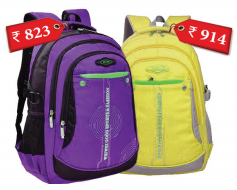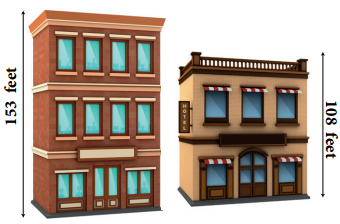Students can download 4th Maths Term 1 Chapter 2 Numbers Ex 2.3c Questions and Answers, Notes, Samacheer Kalvi 4th Maths Guide Pdf helps you to revise the complete Tamilnadu State Board New Syllabus, helps students complete homework assignments and to score high marks in board exams.

## Tamilnadu Samacheer Kalvi 4th Maths Solutions Term 1 Chapter 2 Numbers Ex 2.3c

A. subtract
Question 1.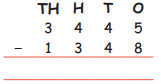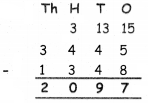Question 2.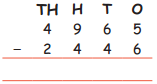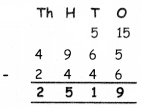Question 3.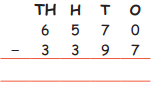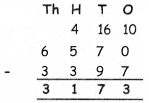Question 4.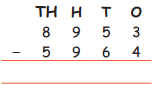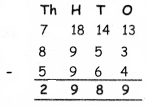B. Find the difference between given numbers.
(a) 4352 and 5020
(b) 1438 and 3370
(c) 2526 and 8431
(d) 3361 and 9000
(a) 4352 and 5020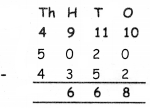(b) 1438 and 3370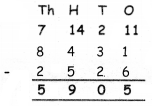(c) 2526 and 8431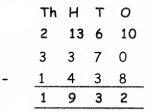(d) 3361 and 9000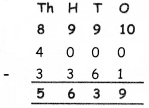Question 1.
The sum of two numbers is 7036, one number is 3168. Find the other number.
sum of two numbers = 7036
one number = 3168
other number = ?
7036 – 3118
= 3868
other number = 3868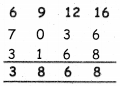Question 2.
A man had Rs 9200 in the bank. He withdrew Rs 2756. How much money does he have in the bank now?
Total amount in a bank = Rs. 9200
Withdraw I = Rs. 2756
Remauning Money = Rs. 6444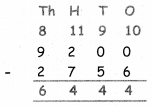D. Create the subtraction story problems for the details given below.

a. 1997 – 1968